Courses

31 Year NEET Questions: Hydrogen

14 Questions MCQ Test Chemistry 31 Years NEET Chapterwise Solved Papers | 31 Year NEET Questions: Hydrogen

Description
Attempt 31 Year NEET Questions: Hydrogen | 14 questions in 30 minutes | Mock test for NEET preparation | Free important questions MCQ to study Chemistry 31 Years NEET Chapterwise Solved Papers for NEET Exam | Download free PDF with solutions
QUESTION: 1

The reaction of H2O2 with H2S is an example of ........reaction 

Solution: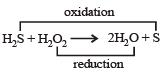In this reaction H2S is oxidised to sulphur and H2O2 is reduced to H2O, hence this reaction show oxidation-reduction both i.e., redox reaction.

QUESTION: 2

Hydrogen peroxide acts both as an oxidising and as a reducing agent depending upon the nature of the reacting species. In which of the following cases H2O2 acts as a reducing agent in acid medium ? 

Solution: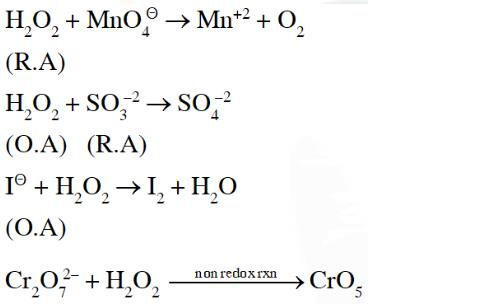QUESTION: 3

Calgon used as a water softener is 

Solution:

The complex salt of metaphosphoric acid sodium hexametaphosphate (NaPO3)6, is known as calgon. It is represented as Na2[Na4(PO3)6]

QUESTION: 4

The ionization of hydrogen atom would give rise to

Solution: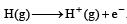QUESTION: 5

At its melting point ice is lighter than water because 

Solution:

In the structure of ice each molecule of H2O is surrounded by three H2O molecules in hexagonal honey comb manner. On the other in water, each molecule is surrounded by four neighbouring molecules randomly which results an open cage like structure. As a result there are a number of 'hole' or open spaces.
In such a structure lesser number of molecules are packed per ml. When ice melts a large no. of hydrogen bonds are broken.
The molecules therefore move into the holes or open spaces and come closer to each other than they were in solid state. This result sharp increase in the density. Therefore ice has lower density than water.

QUESTION: 6

The dielectric constant of H2O is 80. The electrostatic force of attraction between Na+ and Cl will be 

Solution:

Electrostatic forces of attraction are reduced to 1/80th in water.

QUESTION: 7

The O – O – H bond angle in H2O2 is 

Solution:

O – O – H bond angle in H2O2 is 97°

QUESTION: 8

Which of the following groups of ions makes the water hard ? 

Solution:

Temporary hardness is due to presence of bicarbonates of calcium and magnesium and permanent hardness is due to the sulphates or chlorides of both of calcium and magnesium.

QUESTION: 9

The volume strength of 1.5 NH2O2 solution is

Solution:

Volume strength = 5.6 × Normality = 5.6 × 1.5 = 8.4 L

QUESTION: 10

The hydride ion, H, is a stronger base than the hydroxide ion, OH. Which one of the following reactions will occur if sodium hydride (NaH) is dissolved in water? 

Solution: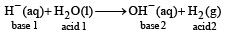In this reaction H acts as bronsted base as it accepts one proton (H+) from H2O and for H2.

OR

In this reaction H−H- accepts H+H+ and as such acts as a base, H2OH2O gives H+H+ and as such acts as an acid.

QUESTION: 11

Which one of the following pairs of substances on reaction will not evolve H2 gas? 

Solution: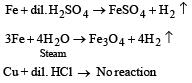Copper does not evolve H2 from acid as it is below hydrogen in electro chemical series.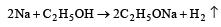QUESTION: 12

When a substance A reacts with water it produces a combustible gas B and a solution of substance C in water. When another substance D reacts with this solution of C, it also produces the same gas B on warming but D can produce gas B on reaction with dilute sulphuric acid at room temperature. A imparts a deep golden yellow colour to a smokeless flame of Bunsen burner. A, B, C and D respectively are 

Solution: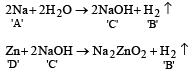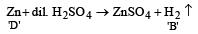Na produces golden yellow color with smokeless flame of Bunsen burner. Yellow is the characteristic color of Sodium.

QUESTION: 13

When H2O2 is oxidised the product is 

Solution: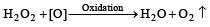QUESTION: 14

Some statements about heavy water are given below:
(a) Heavy water is used as a moderator in nuclear reactors.
(b) Heavy water is more associated than ordinary water.
(c) Heavy water is more effective solvent than ordinary water.
Which of the above statements are correct?                                                                                                 

Solution:

∴ Correct choice : (b)Use Code STAYHOME200 and get INR 200 additional OFF Use Coupon Code

How to Prepare for NEET

Read our guide to prepare for NEET which is created by Toppers & the best Teachers

Track your progress, build streaks, highlight & save important lessons and more!

Similar ContentRelated tests